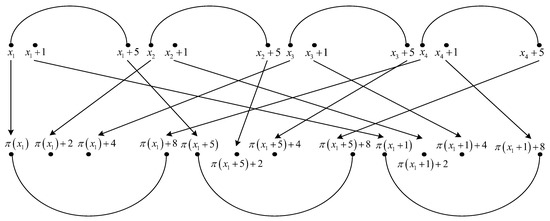Next Article in Journal
Detection of Hypoglycemia Using Measures of EEG Complexity in Type 1 Diabetes Patients
Previous Article in Journal
A History of Thermodynamics: The Missing Manual
Article

# Lengths for Which Fourth Degree PP Interleavers Lead to Weaker Performances Compared to Quadratic and Cubic PP Interleavers

1
Department of Telecommunications and Information Technologies, “Gheorghe Asachi” Technical University, 700506 Iasi, Romania
2
Samsung Electronics, Inc., Suwon 16677, Korea
*
Author to whom correspondence should be addressed.
Entropy 2020, 22(1), 78; https://doi.org/10.3390/e22010078
Received: 25 November 2019 / Revised: 31 December 2019 / Accepted: 6 January 2020 / Published: 8 January 2020
In this paper, we obtain upper bounds on the minimum distance for turbo codes using fourth degree permutation polynomial (4-PP) interleavers of a specific interleaver length and classical turbo codes of nominal 1/3 coding rate, with two recursive systematic convolutional component codes with generator matrix $G = [ 1 , 15 / 13 ]$ . The interleaver lengths are of the form $16 Ψ$ or $48 Ψ$ , where $Ψ$ is a product of different prime numbers greater than three. Some coefficient restrictions are applied when for a prime $p i ∣ Ψ$ , condition $3 ∤ ( p i − 1 )$ is fulfilled. Two upper bounds are obtained for different classes of 4-PP coefficients. For a 4-PP $f 4 x 4 + f 3 x 3 + f 2 x 2 + f 1 x ( mod 16 k L Ψ )$ , $k L ∈ { 1 , 3 }$ , the upper bound of 28 is obtained when the coefficient $f 3$ of the equivalent 4-permutation polynomials (PPs) fulfills $f 3 ∈ { 0 , 4 Ψ }$ or when $f 3 ∈ { 2 Ψ , 6 Ψ }$ and $f 2 ∈ { ( 4 k L − 1 ) · Ψ , ( 8 k L − 1 ) · Ψ }$ , $k L ∈ { 1 , 3 }$ , for any values of the other coefficients. The upper bound of 36 is obtained when the coefficient $f 3$ of the equivalent 4-PPs fulfills $f 3 ∈ { 2 Ψ , 6 Ψ }$ and $f 2 ∈ { ( 2 k L − 1 ) · Ψ , ( 6 k L − 1 ) · Ψ }$ , $k L ∈ { 1 , 3 }$ , for any values of the other coefficients. Thus, the task of finding out good 4-PP interleavers of the previous mentioned lengths is highly facilitated by this result because of the small range required for coefficients $f 4 , f 3$ and $f 2$ . It was also proven, by means of nonlinearity degree, that for the considered inteleaver lengths, cubic PPs and quadratic PPs with optimum minimum distances lead to better error rate performances compared to 4-PPs with optimum minimum distances. View Full-Text
Keywords:
Show FiguresFigure 1

MDPI and ACS Style

Trifina, L.; Tarniceriu, D.; Ryu, J.; Rotopanescu, A.-M. Lengths for Which Fourth Degree PP Interleavers Lead to Weaker Performances Compared to Quadratic and Cubic PP Interleavers. Entropy 2020, 22, 78. https://doi.org/10.3390/e22010078

AMA Style

Trifina L, Tarniceriu D, Ryu J, Rotopanescu A-M. Lengths for Which Fourth Degree PP Interleavers Lead to Weaker Performances Compared to Quadratic and Cubic PP Interleavers. Entropy. 2020; 22(1):78. https://doi.org/10.3390/e22010078

Chicago/Turabian Style

Trifina, Lucian, Daniela Tarniceriu, Jonghoon Ryu, and Ana-Mirela Rotopanescu. 2020. "Lengths for Which Fourth Degree PP Interleavers Lead to Weaker Performances Compared to Quadratic and Cubic PP Interleavers" Entropy 22, no. 1: 78. https://doi.org/10.3390/e22010078

Find Other Styles
Note that from the first issue of 2016, MDPI journals use article numbers instead of page numbers. See further details here.

1